# How to Retrieve Text Before Specific Character in a String in Excel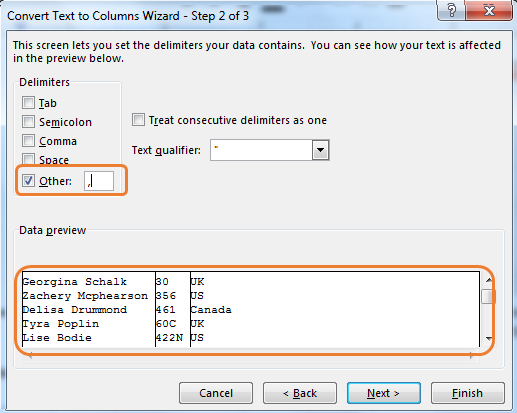In this article, we will learn How to Retrieve Text Before a Specific Character in a String in Excel.

Scenario:

Splitting of text names is one of the basic tasks in excel. We often need to split names, ids, addresses etc. from a cell having all values. To do so we target a separator text in the string and use it to split each segment of string. In this article, we will learn how to split text based on space characters in the string.

Retrieve names using Excel formula in Excel

We want to split texts at space character ( " " ) using the function LEFT, RIGHT, LEN and FIND. There will of course be at least two parts of the name split. First, the text before specific text (text on left of the character) and second, the text after the specific text (text on the right of the character).

Generic formula to get the first name

 =LEFT(cell_ref,FIND(" ", cell_ref)-1)

Cell_ref : reference of the cell where value is stored

Example :

All of these might be confusing to understand. Let's understand how to use the function using an example. Here I have this simple data set. In range A2:A1, we have names and codes concatenated with a comma (",") and space characters (" "). The name is on the left of comma and I want to split it from each text.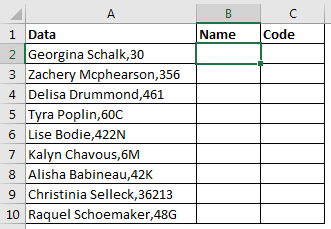Apply the above generic formula here to get text on the left of the comma in string. Copy it in B2 and drag down.

 =LEFT(A2,FIND(",",A2)-1)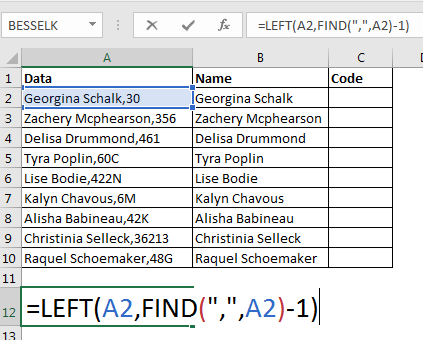You can see that each name is extracted from the string precisely.

As we know, the LEFT function extracts a given number of characters from a given string. Now let's break down the formula inside out.

FIND(",",A2) : Here the FIND function looks for the position of the search string comma (",") in cell A2 (Georgina Schalk,30). It returns 16. Now the formula is =LEFT(A2,16-1).

Since we don't want the comma (",") to be part of the extracted string, we subtract 1 from 16. (if the target characters were 2 then we would subtract 2 and so on. In short, we subtract length of the search string from the position of the search string to not include it in the result).

Finally, we will have LEFT(A2,15) and we get the name extracted (15 characters from left).

Split text on the right of string

We learned how to get text on the left of a specific character. Now, let's see how we can split text on the right of the specific characters.

Generic formula to get the Last name

 =RIGHT(string,LEN(string)-FIND(" ", string))

String: The string from which you want to split text. This can be a hardcoded value, a cell reference, or a formula.

Example:

All of these might be confusing to understand. Let's understand how to use the function using an example. Here We will use the same data that we used in the above example. This time we need to extract codes from the string. The codes are on the right of the comma (","). Let's use the above generic formula to split text from right.

In cell C2, write this formula and drag down to get each value.

 =RIGHT(A2,LEN(A2)-FIND(",",A2))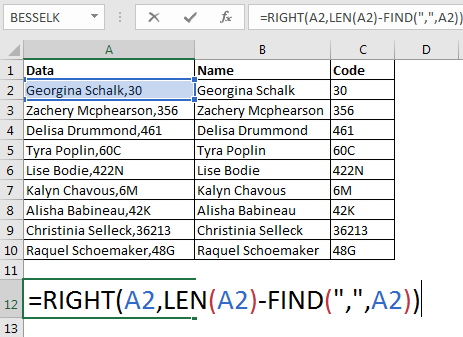Let's break it down from inside. The LEN function returns the number of characters in the string.

LEN(A2) returns 18. The FIND function returns 16, as it does previously. Now the formula solves to  RIGHT(A2,18-16), which again resolves to RIGHT(A2,2). Now as we know, the RIGHT function returns the given number of characters from the right of a given string, hence we get 30 (2 characters from right).

So we have split text at specific text in a string using the text excel function. It is dynamic. Whenever you enter a new data column, you will get extracted text in adjacent columns (if you copy formulas in adjacent cells). Using this method you will have original text intact. If you want, you can paste the output and delete the source data.

Using VBA code to Split text by specific character

Identifying any special characters can be very important for data cleaning purposes. And in some cases, it must be done. So how can we do this in Excel? How can we know if a string contains any special characters? Well we can use User Defined Function in VBA to do so.

The below formula will return TRUE if any cell contains any characters other than 1 to 0 and A to Z (in both cases). If it does not find any special characters it will return FALSE.

Generic Formula

 =Function_name(string)

String: The string that you want to check for special characters.

For this formula to work, you will need to put the below code in a module of your workbook.

Follow the steps as explained. So open Excel. Press ALT+F11 to open the VBA code environment. Insert a module from the Insert menu. Copy the below code and paste it into the module.

 Function Special_Char(str As String) As Boolean For I = 1 To Len(str)  ch = Mid(str, I, 1)  Select Case ch   Case "0" To "9", "A" To "Z", "a" To "z", " "    ContainsSpecialCharacters = False   Case Else    ContainsSpecialCharacters = True   Exit For  End Select Next End Function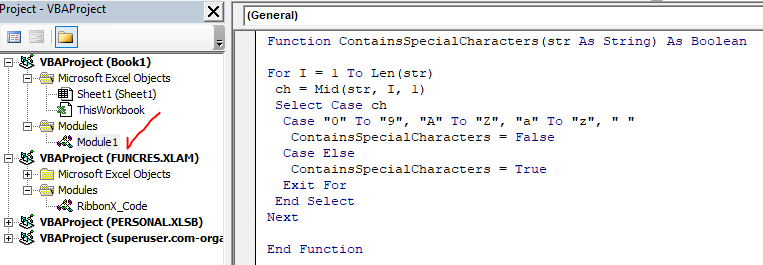Now the function is ready to be used.

Go to the worksheet in the workbook that contains the strings that you want to check.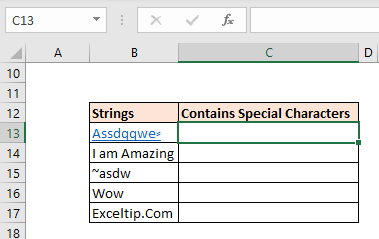Write the below formula in cell C2

 =ContainsSpecialCharacters(B13)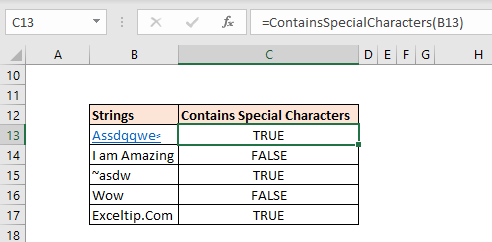It returns TRUE for the first string since it contains a special character. When you copy the formula down it shows FALSE for B14 string and so on.

But strangely it shows TRUE for the last string "Exceltip.com". It is because it contains dot (.). But why so? Let's examine the code to understand.

We are iterating through all the characters of the string using the For loop and mid function of VBA. The Mid function extracts one character at a time from string and stores it into ch variable..

 For I = 1 To Len(str)  ch = Mid(str, I, 1)

Now the main part comes. We use the select case statement to check what the ch variable contains. We tell VBA to check if ch contains any of the defined values. I have defined 0 to 9, A to Z, a to z and " " (space). If c

h contains any of these, we set it's value to False.

 Select Case ch   Case "0" To "9", "A" To "Z", "a" To "z", " "    ContainsSpecialCharacters = False

You can see that we don't have a dot (.) in the list and this is why we get TRUE for the string that contains dot. You can add any character to the list to be exempt from the formula.

If ch contains any character other than the listed characters we set the function's result to True and end the loop right there.

 Case Else    ContainsSpecialCharacters = True Exit For

This will do it

## Text To Column Feature to Split text by specific character

Using the above formula, we can only separate text on the first appearance of specific text in the string. If there were multiple commas at random places in text, the above formula would be useless. We will have to use more formulas and that would be tedious. How do we split text on each appearance of a specific character?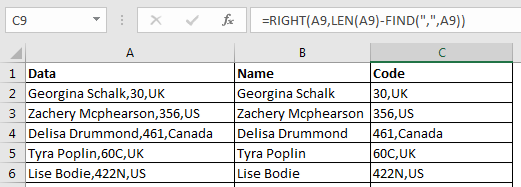There's a built-in feature to split text into columns. It is called Text to Column feature. Using this feature you can split original text into multiple columns, on each appearance of the separator.

Let's see how you can use split text based on a specific character. First thing, have enough empty columns so the right of the source text. If you have data in columns to the right of source text, they may get replaced. It is better to copy the source data in a new sheet. In this way, you will have your source data intact and no other data will be affected.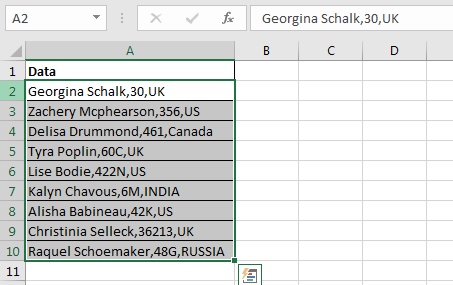Select the data that you want to split.

Press ALT>A>E. This will open the Convert Text to Column Wizard box. You can also go to Data> Text to Column, in the ribbon tab.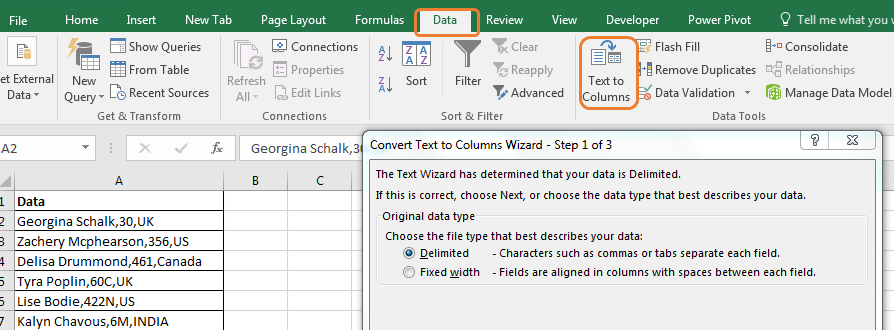Select Delimited and click on next.

There are few common separator options available to select, like Tab, Semicolon, Comma, Space and Other. You can select one or more separators.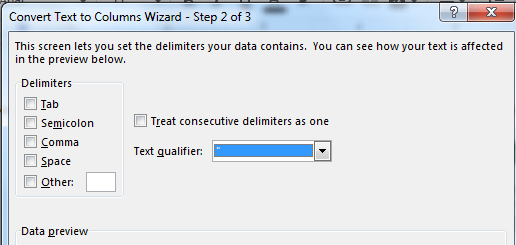Here we will split text using comma separators. We can select the comma check box, but here we will use the Other option. In another option, you can write any text to split text at. We will write a comma.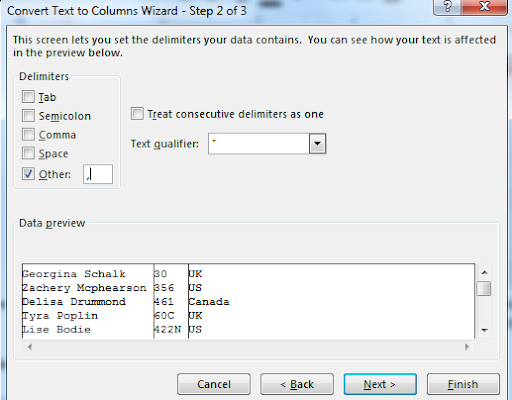Once you write a comma in the text box, excel will preview the output before you finish.Hit the finish button. It's done. Your data is splitted at specified character.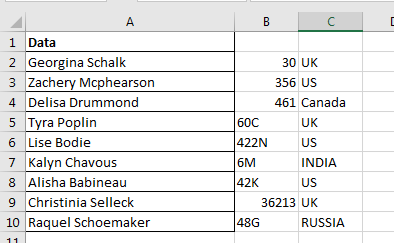If we had used multiple separators, like space and comma both then we would have split first and last names.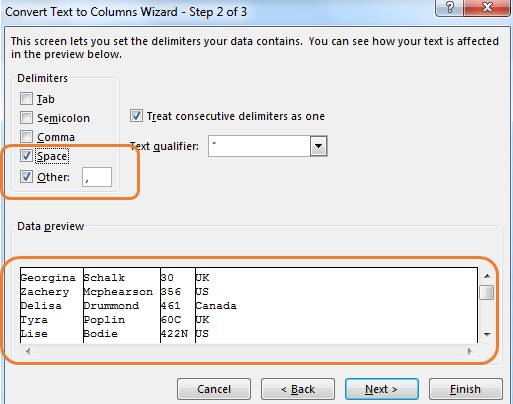In the first method you create a dynamic separator that you can use multiple times by just changing the data. But when you have to split text into too many parts, the Excel formula method can be complex and tedious. On the other hand, the Text to Column method is good on this part but it is not dynamic.

Here are all the observational notes using the formula in Excel
Notes :

1. Select the special character either using directly or using CHAR/CODE function.
2. To get the Middle name just substitute the extracted first name and last name with empty cell (""). This will omit the first name and last name, remaining with the middle name.

Hope this article about How to Retrieve Text Before Specific Character in a String in Excel is explanatory. Find more articles on calculating values and related Excel formulas here. If you liked our blogs, share them with your friends on Facebook. And also you can follow us on Twitter and Facebook. We would love to hear from you, do let us know how we can improve, complement or innovate our work and make it better for you. Write to us at info@exceltip.com.

Related Articles :

Excel REPLACE vs SUBSTITUTE function: The REPLACE and SUBSTITUTE functions are the most misunderstood functions. To find and replace a given text we use the SUBSTITUTE function. Where REPLACE is used to replace a number of characters in a string.

How to use the ISTEXT function in Excel : returns the TRUE logic value if the cell value is text using the ISTEXT function in Excel.

How to Highlight cells that contain specific text in Excel : Highlight cells based on the formula to find the specific text value within the cell in Excel.

Converts decimal Seconds into time format : As we know that time in excel is treated as numbers. Hours, Minutes, and Seconds are treated as decimal numbers. So when we have seconds as numbers, how do we convert into time format? This article got it covered.

Calculate Minutes Between Dates & Time in Excel : calculating the time difference is quite easy. Just need to subtract the start time from the end time. Learn more about this formula clicking the link

Replace text from end of a string starting from variable position : To replace text from the end of the string, we use the REPLACE function. The REPLACE function use the position of text in the string to replace.

Popular Articles :